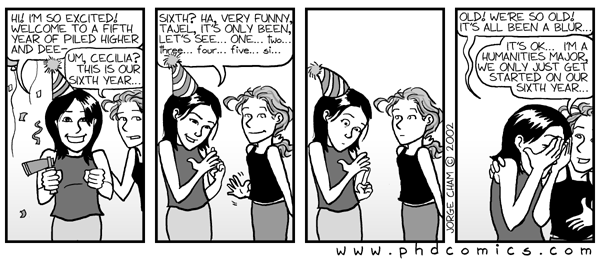# CSCE 782: Problem Set 2

## Distributed Coalition Formation

The goal of this problem set is to implement the find-coalition algorithm (figure 4.7) from the textbook. In order to make the value functions more interesting (realistic) you will do the following.

There are num-skills (slider) available to the agents. Each agent (there are num-agents of them) is born with a my-skills vector which contains a number between 0 a 1 for each one of these skills. These vectors represent how well the agent can perform each one of the skills.

There is also a breed of tasks, of which there are num-tasks. Each task has a requirements variable which is a vector of size num-skill where each entry is a number randomly chosen between 0 and max-need.

We then calculate v(S) for each subset S of agents and each task t by adding all the my-skills vectors for all the agents in S and subtracting that from t.requirements. That is, the closer the resulting vector is to t.requirements the higher the value should be. Note that you will need to take the abs of the difference and multiply by -1. The agent finds the t which maxiximes v(S), for all S, and this is the v(S) that he uses in the find-coalition algorithm.

Given a set of such tasks, you will then implement the find-coalition algorithm to find the set of coalitions that the agents could form.

## Bonus questions

1. How do we visualize this? Maybe you can link the agents to the task they agree do perform. Maybe you can use color to identify affiliations? Maybe use 3D graphics?
2. What can we say about the solution found by this algorithm? Is it in the core? Can we say anything about its quality?
3. What happens if the tasks constantly arrive at some fixed rate and the agents in a coalition require a fixed amount of time to deal with each task? Implement this modification to see how things change.
4. Can you find a better algorithm? How do you define "better"?

### Submission Instructions

As will all the problem sets, you will hand them in using our department's dropbox.José M. Vidal